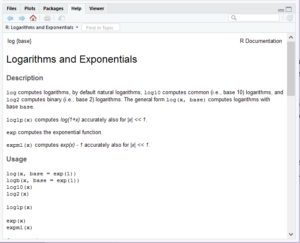Search

# How to Apply Functions: Step Three in Learning R Programming for Free

I hope you are enjoying the “Learning R Programming for Free” series. In the previous (Step 1, Step 2)segments we discussed the R Studio IDE; assigning values to variables; printing the values stored in a variable and the “ls” function; and listing the variables assigned in your workspace.

In this segment, we will introduce some functions and show you how to look at the internals of a function.

We learned in the previous installment that we can assign values to variables, for example:

> pi <- 3.14

Then print the value stored in the variable:

> pi  3.14

We also learned about the “ls()” function.  If we want to see the internals of the “print” or “ls” function, it is as simple as typing the function without the parentheses and expected arguments:> ls function (name, pos = -1L, envir = as.environment(pos), all.names = FALSE, pattern, sorted = TRUE) { if (!missing(name)) { pos <- tryCatch(name, error = function(e) e) if (inherits(pos, “error”)) { name <- substitute(name) if (!is.character(name)) name <- deparse(name) warning(gettextf(“%s converted to character string”, sQuote(name)), domain = NA) pos <- name } } all.names <- .Internal(ls(envir, all.names, sorted)) if (!missing(pattern)) { if ((ll <- length(grep(“[“, pattern, fixed = TRUE))) && ll != length(grep(“]”, pattern, fixed = TRUE))) { if (pattern == “[“) { pattern <- “\\[” warning(“replaced regular expression pattern ‘[‘ by  ‘\\\\[‘”) } else if (length(grep(“[^\\\\]\\[<-“, pattern))) { pattern <- sub(“\\[<-“, “\\\\\\[<-“, pattern) warning(“replaced ‘[<-‘ by ‘\\\\[<-‘ in regular expression pattern”) } } grep(pattern, all.names, value = TRUE) } else all.names } <bytecode: 0x000000000a4c1ae0> <environment: namespace:base>>

Passing arguments is as simple as enclosing the argument in parentheses.  For example, if we want to know the square root of 4, we can write it as follows:

> sqrt(4)  2 To find the square of 2, we use the “^” caret: > 2^2  4 Not to be confused with the “exp()” function which is the function for the mathematical constant “e”: > exp(3)  20.08554 To wrap up this segment, we will discuss the help system in R.  The internal help for any function can be accessed as follows help (<function name>),  For example to get help on exp: > help(exp) Which opens a window in the right Help panel of the IDE:But, help does not work for operators like our caret ^:

> help(^) Error: unexpected ‘^’ in “help(^”

In our next installment, I will discuss data sets that are available for us to practice and learn R.